# 链表合并算法和 HDFS 工作流程# 链表合并算法和HDFS工作流程

## 链表合并算法

### 题目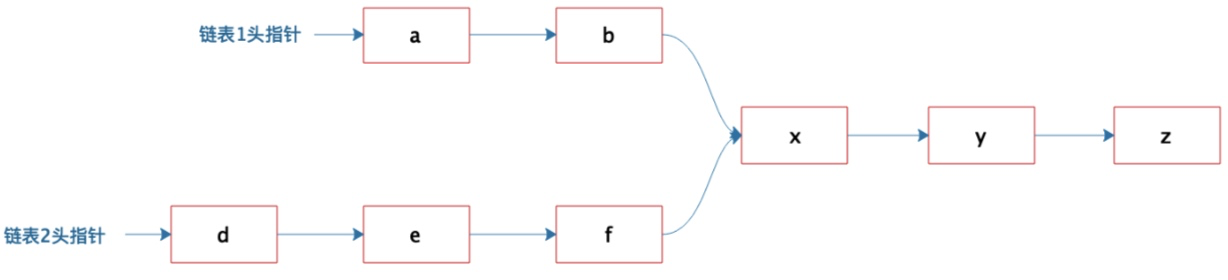﻿

### 题目分析

1. 合并的部分肯定是链表的尾节点到某个节点之间组成的一个子链表。

2. 分别从两个链表的那个节点开始合并需要从头遍历，但是基于上面的分析可知，应该从尾部开始遍历。

3. 由上面两点可知如果链表倒置的话解题就比较容易了，但是题中要求不改变链表，因此需要从头分别遍历两个链表，然后再从两个链表的尾部开始进行对比，因此需要一个后进先出的数据结构存在遍历后的链表结点

4. 从尾部开始比较遇到第一个不相同的元素则终止合并判断

﻿

### 解题流程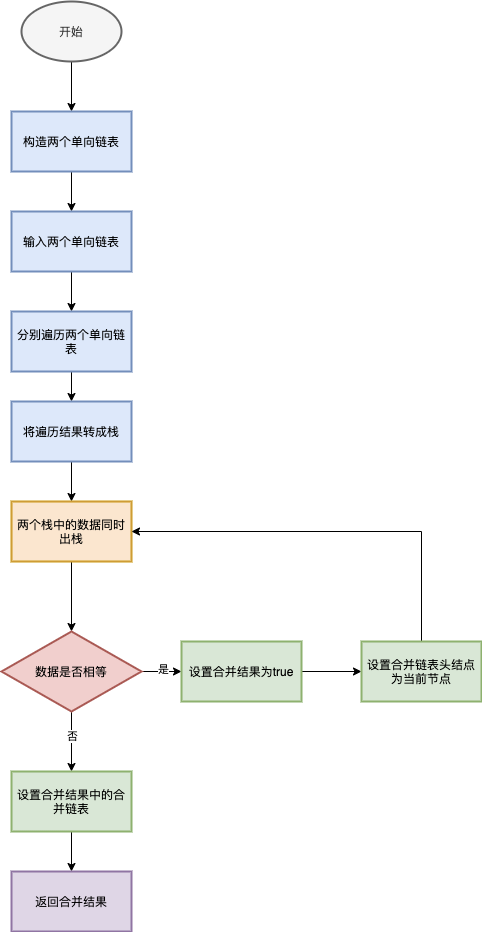﻿

### 转栈实现方法

﻿

public class Linked<E> {    //链表元素数量    transient int size = 0;    //头结点    transient Node<E> first;    //尾结点    transient Node<E> last;﻿    public Linked() {﻿    }﻿    /**     * 多入参构建链表     * @param es     */    @SafeVarargs    public Linked(E... es) {        for (E e:es){            add(e);        }    }﻿    /**     * 添加元素     * @param e     * @return     */    public boolean add(E e) {        final Node<E> l = last;        final Node<E> newNode = new Node<>(e,null);        last = newNode;        if (l == null)            first = newNode;        else            l.next = newNode;        size++;        return true;    }﻿    /**     * 移除元素     * @param e     * @return     */    public boolean remove(E e) {        if(e != null){            //头结点            if(e.equals(first.item)){                Node<E> newNode = first.next;                first.next = null;                this.first = newNode;                size --;                return true;            }            //非头结点            Node<E> pre = first;            for (Node<E> x = first.next; x != null; x = x.next) {                if (e.equals(x.item)) {                    pre.next = x.next;                    x.next = null;                    size --;                    return true;                }                pre = x;            }        }        return false;    }﻿    /**     * 查找节点     * @param e     * @return     */    public Node<E> get(E e) {        if(e != null){            for (Node<E> x = first; x != null; x = x.next) {                if (e.equals(x.item)) {                    return x;                }            }        }        return null;    }﻿    /**     * 获取链表大小     * @return     */    public int size() {        return size;    }﻿    /**     * 链表节点     */    public static class Node<E> {        public E item;        public Node<E> next;        Node(E element, Node<E> next) {            this.item = element;            this.next = next;        }﻿        @Override        public String toString() {            return "Node{" +                    "item=" + item +                    ", next=" + next +                    '}';        }﻿    }﻿    @Override    public String toString() {        if(first == null){            return null;        }        StringBuilder s = new StringBuilder("Linked{");        for (Node<E> x = first; x != null; x = x.next) {            s.append(x.item).append(",");        }        return s.toString().substring(0,s.length()-1) + "}";    }}

﻿

﻿

public class Merge<E> {﻿    /**     * 转栈方法合并两个单项链表     * 时间复杂度O(m+2n) => O(n)     * 空间复杂度O(m+n) => O(n)     * @param linked1     * @param linked2     * @return     */    public Merge.Result<E> merge(Linked<E> linked1, Linked<E> linked2) {        Merge.Result<E> result = new Merge.Result<>();﻿        //1.先将两个单向链表转换成两个栈        Stack<Linked.Node<E>> stack1 = convertToStack(linked1);        Stack<Linked.Node<E>> stack2 = convertToStack(linked2);﻿        //2.将两个栈数据出栈进行比较，如果相同则记录当前节点为新链表的头节点        Linked<E> mergeLinked = new Linked<>();﻿        //最坏情况是时间复杂度O(n),空间复杂度O(n)        Linked.Node<E> e1 = null;        Linked.Node<E> e2 = null;        for(int i=0; i < Math.min(linked1.size,linked2.size); i++){            e1 = stack1.pop();            e2 = stack2.pop();            if(!e1.equals(e2)){                break;            }        }        if(e1 != null && e1.next.equals(e2.next)){            result.setIfMerge(true);            mergeLinked.first = e1.next;            result.setMergeLinked(mergeLinked);        }        return result;    }﻿    /**     * 链表转栈     * 时间复杂度O(n),空间复杂度O(n)     * @param linked     * @return     */    private Stack<Linked.Node<E>> convertToStack(Linked<E> linked) {        if(null == linked){            return null;        }        Stack<Linked.Node<E>> stack = new Stack<>();        for (Linked.Node<E> curr = linked.first; curr != null; curr = curr.next){            stack.add(curr);        }        return stack;    }﻿    /**     * 合并结果     * @author zengdezheng3     * @date 2020-07-24 16:13     */    public static class Result<E> {        private boolean ifMerge;        private Linked<E> mergeLinked;﻿        public boolean isIfMerge() {            return ifMerge;        }﻿        public void setIfMerge(boolean ifMerge) {            this.ifMerge = ifMerge;        }﻿        public Linked<E> getMergeLinked() {            return mergeLinked;        }﻿        public void setMergeLinked(Linked<E> mergeLinked) {            this.mergeLinked = mergeLinked;        }﻿        @Override        public String toString() {            return "Result{" +                    "ifMerge=" + ifMerge +                    ", mergeLinked=" + mergeLinked +                    '}';        }    }

﻿

﻿

    @Test    public void test(){        Linked<String> linked1 = new Linked<>("a","b","x","y","z");        Linked<String> linked2 = new Linked<>("d","e","f","x","y","z");        Merge<String> merge = new Merge<>();        Merge.Result<String> result = merge.merge(linked1,linked2);        System.out.println(result.toString());    }

﻿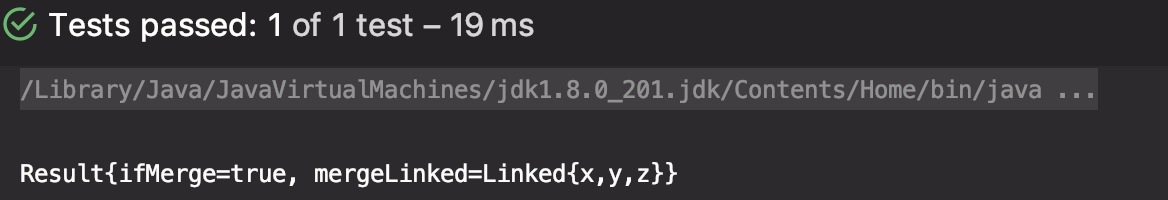﻿

### 跳跃指针解法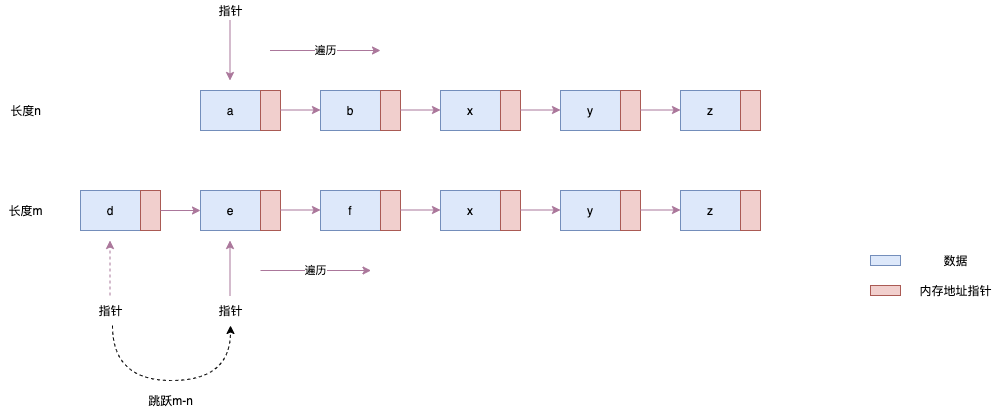﻿

﻿

        @Override        public boolean equals(Object o) {            if (this == o) return true;            if (o == null || getClass() != o.getClass()) return false;            Node<?> node = (Node<?>) o;            return Objects.equals(item, node.item) &&                    Objects.equals(next, node.next);        }

﻿

    /**     * 跳跃指针方法合并两个单项链表     *  时间复杂度O(m)     *  空间复杂度O(n)     * @param linked1     * @param linked2     * @return     */    public Merge.Result<E> mergeByJumpIndex(Linked<E> linked1, Linked<E> linked2) {        //链表1的长度大于等于链表2的长度        if(linked1.size() - linked2.size() >= 0){            return jumpIndex(linked1, linked2);        }else {            return jumpIndex(linked2, linked1);        }    }     /**     * 长短链表跳跃指针合并     * 时间复杂度O(m)     * 空间复杂度O(n)     * @param longLinked     * @param shortLinked     * @return     */    private Merge.Result<E> jumpIndex(Linked<E> longLinked, Linked<E> shortLinked) {        Merge.Result<E> result = new Merge.Result<>();        //长链表的长度        int m = longLinked.size();        //短链表的长度        int n = shortLinked.size();        Linked.Node<E> curr1 = longLinked.first;        //链表1指针跳跃m-n次        for(int i=0;i< m-n;i++){            curr1 = curr1.next;        }        Linked.Node<E> curr2 = shortLinked.first;        for(int i=0;i< n;i++){            if(curr1.equals(curr2)){                Linked<E> mergeLinked = new Linked<>();                mergeLinked.first = curr1;                result.setIfMerge(true);                result.setMergeLinked(mergeLinked);                return result;            }            curr1 = curr1.next;            curr2 = curr2.next;        }        return result;    }

﻿

@Test    public void test1(){        Linked<String> linked1 = new Linked<>("a","b","x","y","z");        Linked<String> linked2 = new Linked<>("d","e","f","x","y","z");        Merge<String> merge = new Merge<>();        Merge.Result<String> result = merge.mergeByJumpIndex(linked1,linked2);        System.out.println("test3 result = " + result.toString());    }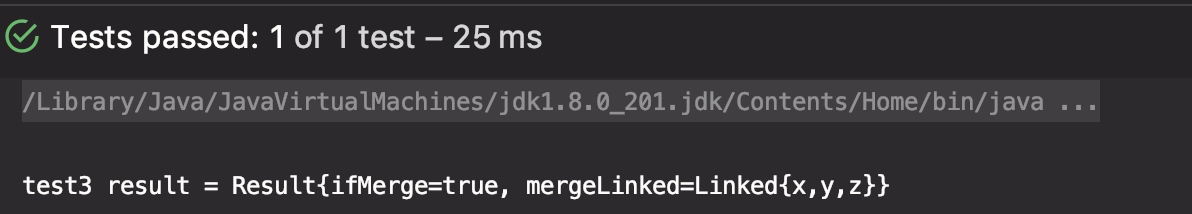﻿

### 合并链表比较法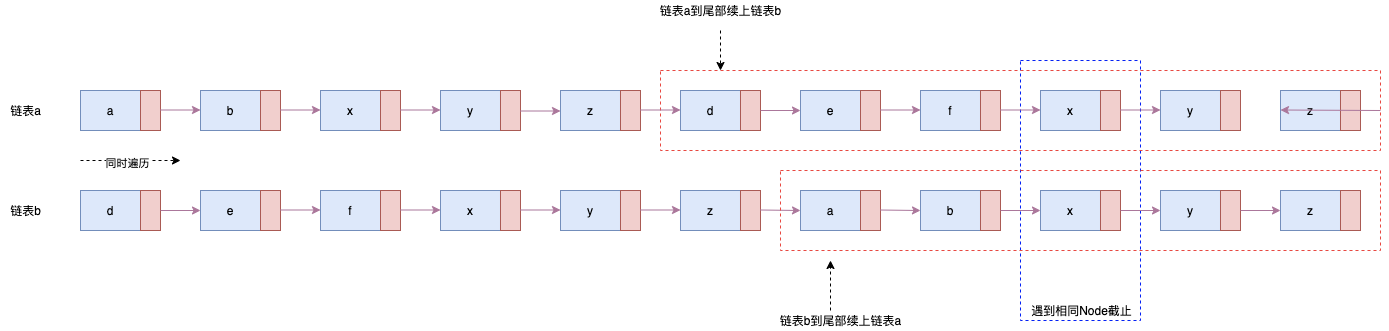﻿

﻿

        @Override        public boolean equals(Object o) {            if (this == o) return true;            if (o == null || getClass() != o.getClass()) return false;            Node<?> node = (Node<?>) o;            return Objects.equals(item, node.item) &&                    Objects.equals(next, node.next);        }

﻿

    /**     * 组合链表比较判断合并     * 时间复杂度O(m+n),空间复杂度O(1)     * @param linked1     * @param linked2     * @return     */    public Merge.Result<E> combination(Linked<E> linked1, Linked<E> linked2) {        Merge.Result<E> result = new Merge.Result<>();        Linked.Node<E> node1 = linked1.first;        Linked.Node<E> node2 = linked2.first;        while (!node1.equals(node2)){            node1 = node1.next == null?linked2.first:node1.next;            node2 = node2.next == null?linked1.first:node2.next;        }        if(node1.equals(node2)){            Linked<E> mergeLinked = new Linked<>();            mergeLinked.first = node1;            result.setMergeLinked(mergeLinked);            result.setIfMerge(true);            return result;        }        return result;    }

﻿

    @Test    public void test2(){        Linked<String> linked1 = new Linked<>("a","b","x","y","z");        Linked<String> linked2 = new Linked<>("d","e","f","x","y","z");        Merge<String> merge = new Merge<>();        Merge.Result<String>result = merge.combination(linked1,linked2);        System.out.println("test2 result = " + result.toString());    }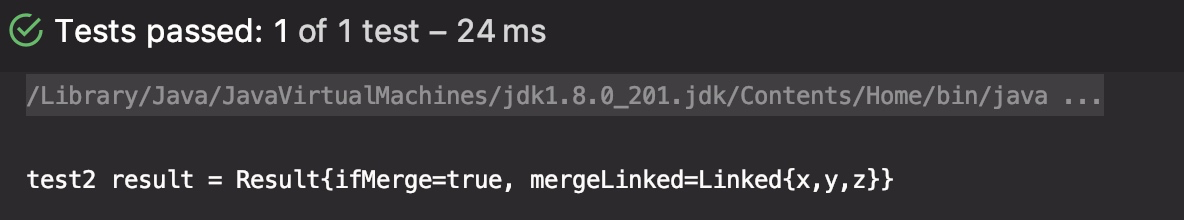﻿

## HDFS工作流程

﻿

### HDFS设计原则

#### 设计目标

• 存储非常大的文件。通常HDFS文件量级是几百M、G、或者TB级别，实际上已经达到了PB级别

• 采用流式的数据访问方式。流式读取最小化了硬盘的寻址开销，只需要寻址一次，然后就一直连续读取数据

• 运行于商业硬件上。HDFS运行在普通廉价的普通服务器上

﻿

#### 不适用范围

• 低延时的数据访问。HDFS是为高吞吐数据传输设计的,因此可能牺牲延时，因此对延时要求在毫秒级别的应用不适用。

• 大量小文件。文件的元数据保存在NameNode的内存中， 整个文件系统的文件数量会受限于NameNode的内存大小，如果存储大量小文件会占据大量内存。

• 多方读写，需要任意的文件修改 。HDFS采用追加（append-only）的方式写入数据。不支持文件任意offset的修改。不支持多个写入器（writer）。

﻿

### 核心概念

#### Namenode

Namenode存放文件系统树及所有文件、目录的元数据。元数据持久化为2种形式：

• namespcae image

• edit log

﻿

﻿

### 工作流程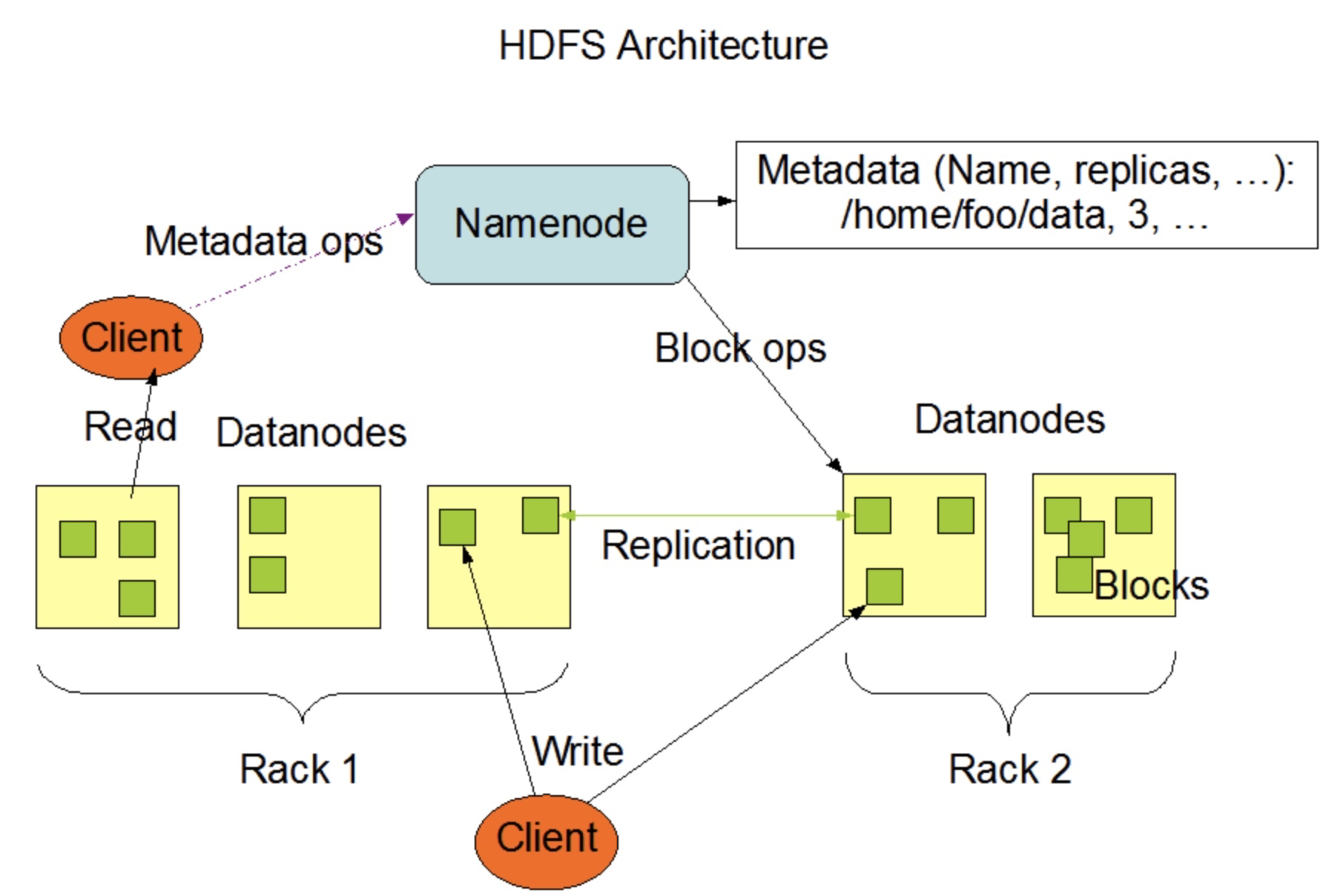﻿

#### 注册流程

DataNode启动后会向NameNode节点发出注册请求，NameNode会将注册结果信息返回给DataNode节点

﻿

﻿

#### DataNode故障流程

﻿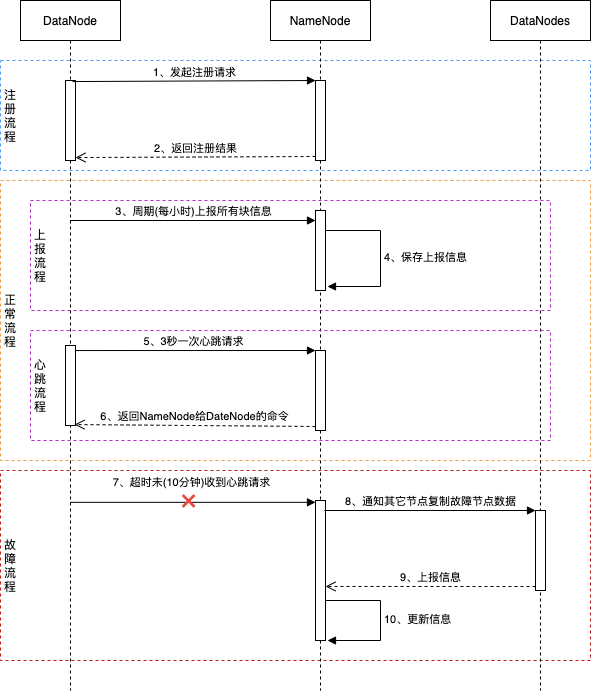﻿## 评论